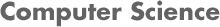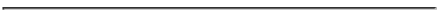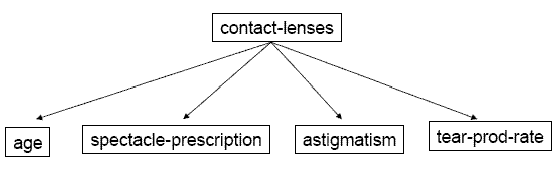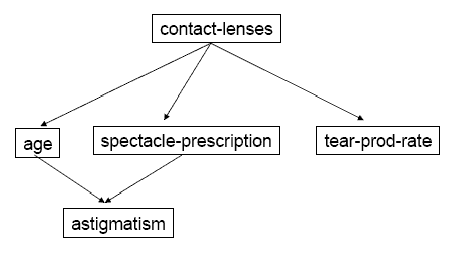### CS534 Artificial Intelligence Homework 8 - Fall 2007

#### PROF. CAROLINA RUIZ

Due Date: At the beginning of class on Tuesday December 4, 2007• Read Sections 18.1-18.3, 20.2, and 20.5 of the textbook.
Sections 18.4-18.5, 20.3-20.4 are encoraged but not required reading.

• Problems: You need to turn in written solutions to all the problems in Part II below. Solve the problems in Part I before the class also so that you can ask questions about them in preparation for Exam 2. Remember to show your work and explain your answers.

1. Part I (all problems in Part I are optional):

• Chapter 18: 18.4, 18.7, 18.8, 18.10, 18.12, 18.13.

• Chapter 20: 20.11, 20.13, 20.15, 20.18.

Hint (18.8):

• Part (a): There are two possible classifications that the leaf can output: either "positive" for all examples in the leaf node, or "negative" for all examples in the leaf node. Compare the two of them to determine which one will produce the minimum absolute error. In this case, the absolute error is the number of examples in the leaf node that are misclassified.
• Part (b): Now we are not restricted to output "positive" or "negative". Instead, we'll think of a positive example as being classified as "1", a negative example as being classified as "0", and the leaf will output a value c, which is a constant, 0 ≤ c ≤ 1, such that this constant minimizes the sum, over all the examples in the leaf, of the values:
(correct classification of the example - c)2.
Find the value of c that minimizes this sum.

2. *Part II (all problems in Part II are required):

1. Decision Trees: (35 points)

Consider the following subset of the Contact Lenses Dataset:

```ATTRIBUTES:	POSSIBLE VALUES:
age             {young,pre-presbyopic,presbyopic}
astigmatism     {no,yes}
tear-prod-rate  {reduced,normal}
contact-lenses  {soft,hard,none} <- classification target
```
 age astigmatism tear-prod-rate contact-lenses young no normal soft young yes reduced none young yes normal hard pre-presbyopic no reduced none pre-presbyopic no normal soft pre-presbyopic yes normal hard pre-presbyopic yes normal none pre-presbyopic yes normal none presbyopic no reduced none presbyopic no normal none presbyopic yes reduced none presbyopic yes normal hard

• (30 points) Construct the full decision tree for the above Contact Lenses dataset where contact-lenses is the (classification) target attribute. Show all the steps of the calculations and of the construction of your decision tree. For your convenience, the logarithm in base 2 of selected values are provided.

 x 1/2 1/3 1/4 3/4 1/5 2/5 3/5 1/6 5/6 1/7 2/7 3/7 4/7 1 log2(x) -1 -1.5 -2 -0.4 -2.3 -1.3 -0.7 -2.5 -0.2 -2.8 -1.8 -1.2 -0.8 0
```
Unrelated example of decision tree construction

(5 points) Use the decision tree you constructed to predict the classification
(contact-lenses) value of the following test instances.
Compute the accuracy of the model on these test data instances.

young,      no,  reduced, none     your decision tree predicts: ______
pre-pre,    yes, reduced, none     your decision tree predicts: ______
presbyopic, no,  normal,  soft     your decision tree predicts: ______
presbyopic, yes, normal,  hard     your decision tree predicts: ______

```

2. Naive Bayes Models: (35 points)

Consider the full Contact Lenses Dataset (24 instances):

```ATTRIBUTES:	         POSSIBLE VALUES:
age                      {young,pre-presbyopic,presbyopic}
spectacle-prescription:  {myope,hypermetrope}
astigmatism              {no,yes}
tear-prod-rate           {reduced,normal}
contact-lenses           {soft,hard,none} <- classification target
```
 age spectacle-prescription astigmatism tear-prod-rate contact-lenses young myope no reduced none young myope no normal soft young myope yes reduced none young myope yes normal hard young hypermetrope no reduced none young hypermetrope no normal soft young hypermetrope yes reduced none young hypermetrope yes normal hard pre-presbyopic myope no reduced none pre-presbyopic myope no normal soft pre-presbyopic myope yes reduced none pre-presbyopic myope yes normal hard pre-presbyopic hypermetrope no reduced none pre-presbyopic hypermetrope no normal soft pre-presbyopic hypermetrope yes reduced none pre-presbyopic hypermetrope yes normal none presbyopic myope no reduced none presbyopic myope no normal none presbyopic myope yes reduced none presbyopic myope yes normal hard presbyopic hypermetrope no reduced none presbyopic hypermetrope no normal soft presbyopic hypermetrope yes reduced none presbyopic hypermetrope yes normal none

• (30 points) Construct the Naive Bayes classifier over this full dataset. That is, compute all P(A=a | contact-lenses=v) for all predicting attributes A, all attribute A's values a, and all contact-lenses values v. Show all the steps of the calculations.

Important:Remember to add 1 to all the counts to avoid the problem of having a probability that is equal to 0. For example, note that the number of instances that have astigmatism=yes among the instances that have contact-lenses=soft is equal to 0. Adding 1 to all the counts means that this count [i.e., count (astigmatism=yes | contact-lenses=soft) ] will become 1. Similarly, count(astigmatism=no | contact-lenses=soft) will be 5 + 1 = 6.

In other words, you need to construct all the conditional probability tables for the Naive Bayes net below based on the 24 data instances:• (5 points) Use the Naive Bayes model you constructed to predict the classification (contact-lenses) value of the following test instances. Compute the accuracy of the model on these test data instances. Show your work.
```young,      myope,        no,  reduced, none     your Naive Bayes model predicts: ______
presbyopic, hypermetrope, yes, normal,  hard     your Naive Bayes model predicts: ______

```

3. Bayesian Net Models: (35 points)

Consider the full Contact Lenses Dataset above (24 instances).

• (30 points) Construct all the conditional probability tables for the Bayesian net below based on the 24 data instances:• (5 points) Use the Bayesian model you constructed to predict the classification (contact-lenses) value of the following test instances. Compute the accuracy of the model on these test data instances. Show your work.
```ASTIGMATISM	TEAR-PROD-RATE	CONTACT-LENSES
no,  		reduced, 	none     	your Bayes net predicts: ______
yes, 		normal,  	hard     	your Bayes net predicts: ______

```